xTorsion (mechanics)Encyclopedia
In solid mechanics
Solid mechanics
Solid mechanics is the branch of mechanics, physics, and mathematics that concerns the behavior of solid matter under external actions . It is part of a broader study known as continuum mechanics. One of the most common practical applications of solid mechanics is the Euler-Bernoulli beam equation...

, torsion is the twisting of an object due to an applied torque
Torque
Torque, moment or moment of force , is the tendency of a force to rotate an object about an axis, fulcrum, or pivot. Just as a force is a push or a pull, a torque can be thought of as a twist....

. In sections perpendicular to the torque axis, the resultant shear stress
Shear stress
A shear stress, denoted \tau\, , is defined as the component of stress coplanar with a material cross section. Shear stress arises from the force vector component parallel to the cross section...

in this section is perpendicular to the radius.

For solid shafts of uniform circular cross-section or hollow circular shafts with constant wall thickness, the torsion relations are: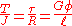where:
• R is the outer radius of the shaft i.e. m, ft.
•is the maximum shear stress at the outer surface.
• φ is the angle of twist in radian
Radian is the ratio between the length of an arc and its radius. The radian is the standard unit of angular measure, used in many areas of mathematics. The unit was formerly a SI supplementary unit, but this category was abolished in 1995 and the radian is now considered a SI derived unit...

s.
• T is the torque (N·m
Newton metre
A newton metre is a unit of torque in the SI system. The symbolic form is N m or N·m, and sometimes hyphenated newton-metre...

or ft·lbf
Foot-pound force
The foot-pound force, or simply foot-pound is a unit of work or energy in the Engineering and Gravitational Systems in United States customary and Imperial units of measure. It is the energy transferred on applying a force of 1 pound-force through a displacement of 1 foot...

).
• is the length of the object the torque is being applied to or over.
• G is the shear modulus or more commonly the modulus of rigidity and is usually given in gigapascals (GPa), lbf/in2
Pounds per square inch
The pound per square inch or, more accurately, pound-force per square inch is a unit of pressure or of stress based on avoirdupois units...

(psi), or lbf/ft2.
• J is the torsion constant
Torsion constant
The torsion constant is a geometrical property of a beam's cross-section which is involved in the relationship between angle of twist and applied torque along the axis of the bar, for a homogeneous linear-elastic bar. That is, the torsion constant describes a beam's torsional stiffness.- History...

for the section. It is identical to the polar moment of inertia
Polar moment of inertia
Polar moment of inertia is a quantity used to predict an object's ability to resist torsion, in objects with an invariant circular cross section and no significant warping or out-of-plane deformation. It is used to calculate the angular displacement of an object subjected to a torque...

for a round shaft or concentric tube only. For other shapes J must be determined by other means. For solid shafts the membrane analogy
Membrane analogy
The elastic membrane analogy, also known as the soap-film analogy, was first published by pioneering aerodynamicist Ludwig Prandtl in 1903....

is useful, and for thin walled tubes of arbitrary shape the shear flow approximation is fairly good, if the section is not re-entrant. For thick walled tubes of arbitrary shape there is no simple solution, and finite element analysis
Finite element method
The finite element method is a numerical technique for finding approximate solutions of partial differential equations as well as integral equations...

(FEA) may be the best method.
• The product GJ is called the torsional rigidity.

The shear stress at a point within a shaft is: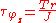where:
• r is the distance from the center of rotation

Note that the highest shear stress is at the point where the radius is maximum, the surface of the shaft. High stresses at the surface may be compounded by stress concentrations such as rough spots. Thus, shafts for use in high torsion are polished to a fine surface finish to reduce the maximum stress in the shaft and increase its service life.

The angle of twist can be found by using: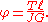## Polar moment of inertia

The polar moment of inertia for a solid shaft is: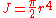where r is the radius of the object.

The polar moment of inertia for a pipe is: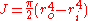where the o and i subscripts stand for the outer and inner radius
In classical geometry, a radius of a circle or sphere is any line segment from its center to its perimeter. By extension, the radius of a circle or sphere is the length of any such segment, which is half the diameter. If the object does not have an obvious center, the term may refer to its...

of the pipe.

For a thin walled cylinder
J = 2π R3 t

where R is the average of the outer and inner radius
and t is the wall thickness.

## Failure mode

The shear stress in the shaft may be resolved into principal stresses via Mohr's circle. If the shaft is loaded only in torsion then one of the principal stresses will be in tension and the other in compression. These stresses are oriented at a 45-degree helical angle around the shaft. If the shaft is made of brittle
Brittle
A material is brittle if, when subjected to stress, it breaks without significant deformation . Brittle materials absorb relatively little energy prior to fracture, even those of high strength. Breaking is often accompanied by a snapping sound. Brittle materials include most ceramics and glasses ...

material then the shaft will fail by a crack initiating at the surface and propagating through to the core of the shaft fracturing in a 45-degree angle helical shape. This is often demonstrated by twisting a piece of blackboard chalk between one's fingers.

• Structural rigidity
Structural rigidity
In discrete geometry and mechanics, structural rigidity is a combinatorial theory for predicting the flexibility of ensembles formed by rigid bodies connected by flexible linkages or hinges.-Definitions:...

• Torsion spring
Torsion spring
A torsion spring is a spring that works by torsion or twisting; that is, a flexible elastic object that stores mechanical energy when it is twisted. The amount of force it exerts is proportional to the amount it is twisted. There are two types...

or -bar
• Torsion siege engine
• Torsional vibration
Torsional vibration
Torsional vibration is angular vibration of an object—commonly a shaft along its axis of rotation. Torsional vibration is often a concern in power transmission systems using rotating shafts or couplings where it can cause failures if not controlled....

• Torque tester
Torque tester
A torque tester is used as a quality control device to test or calibrate torque controlled tools. This includes electronic torque wrenches, click torque wrenches, dial torque wrenches, electric screwdrivers, air screwdrivers, pulse tools, cordless screwdrivers, nutrunners, and torque screwdrivers...

• Saint-Venant's theorem
Saint-Venant's theorem
In solid mechanics, it is common to analyze the properties of beams with constant cross section. Saint-Venant's theorem states that the simply connected cross section with maximal torsional rigidity is a circle...

• List of moments of inertia